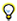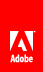# Vector class versus Array classUse the Vector class instead of the Array class, when possible. The Vector class allows faster read and write access than the Array class. A simple benchmark shows the benefits of the Vector class over the Array class. The following code shows a benchmark for the Array class: ```var coordinates:Array = new Array(); var started:Number = getTimer(); for (var i:int = 0; i< 300000; i++) { coordinates[i] = Math.random()*1024; }   trace(getTimer() - started); // output: 107 ``` The following code shows a benchmark for the Vector class: ```var coordinates:Vector. = new Vector.(); var started:Number = getTimer(); for (var i:int = 0; i< 300000; i++) { coordinates[i] = Math.random()*1024; } trace(getTimer() - started); // output: 72 ``` The example can be further optimized by assigning a specific length to the vector and setting its length to fixed: ```// Specify a fixed length and initialize its length var coordinates:Vector. = new Vector.(300000, true); var started:Number = getTimer(); for (var i:int = 0; i< 300000; i++) { coordinates[i] = Math.random()*1024; } trace(getTimer() - started); // output: 48 ``` If the size of the vector is not specified ahead of time, the size increases when the vector runs out of space. Each time the size of the vector increases, a new block of memory is allocated. The current content of the vector is copied into the new block of memory. This extra allocation and copying of data hurts performance. The above code is optimized for performance by specifying the initial size of the vector. However, the code is not optimized for maintainability. To also improve maintainability, store the reused value in a constant: ```// Store the reused value to maintain code easily const MAX_NUM:int = 300000; var coordinates:Vector. = new Vector.(MAX_NUM, true); var started:Number = getTimer(); for (var i:int = 0; i< MAX_NUM; i++) { coordinates[i] = Math.random()*1024; } trace(getTimer() - started); // output: 47 ``` Try to use the Vector object APIs, when possible, as they are likely to run faster.Twitter™ and Facebook posts are not covered under the terms of Creative Commons.// Ethnio survey code removed